JMSLTM Numerical Library 7.2.0
com.imsl.math

## Class MinConGenLin

• All Implemented Interfaces:
Serializable, Cloneable

```public class MinConGenLin
extends Object
implements Serializable, Cloneable```
Minimizes a general objective function subject to linear equality/inequality constraints.

The class `MinConGenLin` is based on M.J.D. Powell's TOLMIN, which solves linearly constrained optimization problems, i.e., problems of the form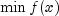subject to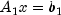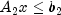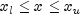given the vectors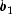,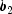,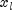, andand the matrices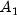and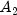.

The algorithm starts by checking the equality constraints for inconsistency and redundancy. If the equality constraints are consistent, the method will revise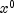, the initial guess, to satisfyNext,is adjusted to satisfy the simple bounds and inequality constraints. This is done by solving a sequence of quadratic programming subproblems to minimize the sum of the constraint or bound violations.

Now, for each iteration with a feasible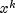, let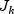be the set of indices of inequality constraints that have small residuals. Here, the simple bounds are treated as inequality constraints. Let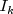be the set of indices of active constraints. The following quadratic programming problem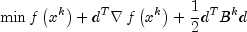subject to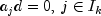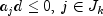is solved to get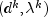where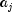is a row vector representing either a constraint inoror a bound constraint on x. In the latter case, the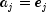for the bound constraint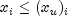and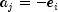for the constraint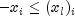. Here,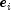is a vector with 1 as the i-th component, and zeros elsewhere. Variables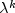are the Lagrange multipliers, and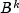is a positive definite approximation to the second derivative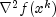.

After the search direction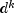is obtained, a line search is performed to locate a better point. The new point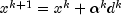has to satisfy the conditions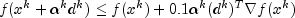and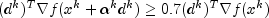The main idea in forming the setis that, if any of the equality constraints restricts the step-length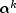, then its index is not in. Therefore, small steps are likely to be avoided.

Finally, the second derivative approximation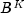, is updated by the BFGS formula, if the condition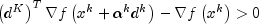holds. Let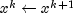, and start another iteration.

The iteration repeats until the stopping criterion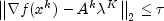is satisfied. Hereis the supplied tolerance. For more details, see Powell (1988, 1989).

Example 1, Example 2, Serialized Form
• ### Nested Class Summary

Nested Classes
Modifier and Type Class and Description
`static class ` `MinConGenLin.ConstraintsInconsistentException`
The equality constraints are inconsistent.
`static class ` `MinConGenLin.ConstraintsNotSatisfiedException`
No vector x satisfies all of the constraints.
`static class ` `MinConGenLin.EqualityConstraintsException`
the variables are determined by the equality constraints.
`static interface ` `MinConGenLin.Function`
Public interface for the user-supplied function to evaluate the function to be minimized.
`static interface ` `MinConGenLin.Gradient`
Public interface for the user-supplied function to compute the gradient.
`static class ` `MinConGenLin.VarBoundsInconsistentException`
The equality constraints and the bounds on the variables are found to be inconsistent.
• ### Constructor Summary

Constructors
Constructor and Description
```MinConGenLin(MinConGenLin.Function fcn, int nvar, int ncon, int neq, double[] a, double[] b, double[] lowerBound, double[] upperBound)```
Constructor for `MinConGenLin`.
• ### Method Summary

Methods
Modifier and Type Method and Description
`int[]` `getFinalActiveConstraints()`
Returns the indices of the final active constraints.
`int` `getFinalActiveConstraintsNum()`
Returns the final number of active constraints.
`double[]` `getLagrangeMultiplierEst()`
Returns the Lagrange multiplier estimates of the final active constraints.
`int` `getNumberOfThreads()`
Returns the number of `java.lang.Thread` instances used for parallel processing.
`double` `getObjectiveValue()`
Returns the value of the objective function.
`double[]` `getSolution()`
Returns the computed solution.
`void` `setGuess(double[] guess)`
Sets an initial guess of the solution.
`void` `setNumberOfThreads(int numberOfThreads)`
Sets the number of `java.lang.Thread` instances to be used for parallel processing.
`void` `setTolerance(double tolerance)`
Sets the nonnegative tolerance on the first order conditions at the calculated solution.
`void` `solve()`
Minimizes a general objective function subject to linear equality/inequality constraints.
• ### Methods inherited from class java.lang.Object

`clone, equals, finalize, getClass, hashCode, notify, notifyAll, toString, wait, wait, wait`
• ### Constructor Detail

• #### MinConGenLin

```public MinConGenLin(MinConGenLin.Function fcn,
int nvar,
int ncon,
int neq,
double[] a,
double[] b,
double[] lowerBound,
double[] upperBound)```
Constructor for `MinConGenLin`.
Parameters:
`fcn` - A `Function` object, user-supplied function to evaluate the function to be minimized.
`nvar` - An `int` scalar containing the number of variables.
`ncon` - An `int` scalar containing the number of linear constraints (excluding simple bounds).
`neq` - An `int` scalar containing the number of linear equality constraints.
`a` - A `double` array containing the equality constraint gradients in the first neq rows followed by the inequality constraint gradients. `a.length` = `ncon` * `nvar`
`b` - A `double` array containing the right-hand sides of the linear constraints.
`lowerBound` - A `double` array containing the lower bounds on the variables. Choose a very large negative value if a component should be unbounded below or set `lowerBound[i] = upperBound[i]` to freeze the i-th variable. `lowerBound.length` = `nvar`
`upperBound` - A `double` array containing the upper bounds on the variables. Choose a very large positive value if a component should be unbounded above. `upperBound.length` = `nvar`
Throws:
`IllegalArgumentException` - is thrown if the dimensions of `nvar`, `ncon`, `neq`, `a.length` , `b.length`, `lowerBound.length` and `upperBound.length` are not consistent.
• ### Method Detail

• #### getFinalActiveConstraints

`public int[] getFinalActiveConstraints()`
Returns the indices of the final active constraints.
Returns:
an `int` array containing the indices of the final active constraints.
• #### getFinalActiveConstraintsNum

`public int getFinalActiveConstraintsNum()`
Returns the final number of active constraints.
Returns:
an `int` scalar containing the final number of active constraints.
• #### getLagrangeMultiplierEst

`public double[] getLagrangeMultiplierEst()`
Returns the Lagrange multiplier estimates of the final active constraints.
Returns:
a `double` array containing the Lagrange multiplier estimates of the final active constraints.

`public int getNumberOfThreads()`
Returns the number of `java.lang.Thread` instances used for parallel processing.
Returns:
an `int` containing the number of `java.lang.Thread` instances used for parallel processing.
• #### getObjectiveValue

`public double getObjectiveValue()`
Returns the value of the objective function.
Returns:
a `double` scalar containing the value of the objective function.
• #### getSolution

`public double[] getSolution()`
Returns the computed solution.
Returns:
a `double` array containing the computed solution.
• #### setGuess

`public void setGuess(double[] guess)`
Sets an initial guess of the solution.
Parameters:
`guess` - a `double` array containing an initial guess.

`public void setNumberOfThreads(int numberOfThreads)`
Sets the number of `java.lang.Thread` instances to be used for parallel processing.
Parameters:
`numberOfThreads` - an `int` specifying the number of `java.lang.Thread` instances to be used for parallel processing. If `numberOfThreads` is greater than 1, then interface `Function.f` is evaluated in parallel and `Function.f` must be thread-safe. Otherwise, unexpected behavior can occur.

Default: `numberOfThreads` = 1.

• #### setTolerance

`public void setTolerance(double tolerance)`
Sets the nonnegative tolerance on the first order conditions at the calculated solution.
Parameters:
`tolerance` - a `double` scalar containing the tolerance.
• #### solve

```public final void solve()
throws MinConGenLin.ConstraintsInconsistentException,
MinConGenLin.VarBoundsInconsistentException,
MinConGenLin.ConstraintsNotSatisfiedException,
MinConGenLin.EqualityConstraintsException```
Minimizes a general objective function subject to linear equality/inequality constraints.
Throws:
`MinConGenLin.ConstraintsInconsistentException`
`MinConGenLin.VarBoundsInconsistentException`
`MinConGenLin.ConstraintsNotSatisfiedException`
`MinConGenLin.EqualityConstraintsException`
JMSLTM Numerical Library 7.2.0